# 978-0073382395 Chapter 18 Questions and Problems 1-12

Document Type
Homework Help
Book Title
Fundamentals of Corporate Finance Standard Edition 9th Edition
Authors
Stephen Ross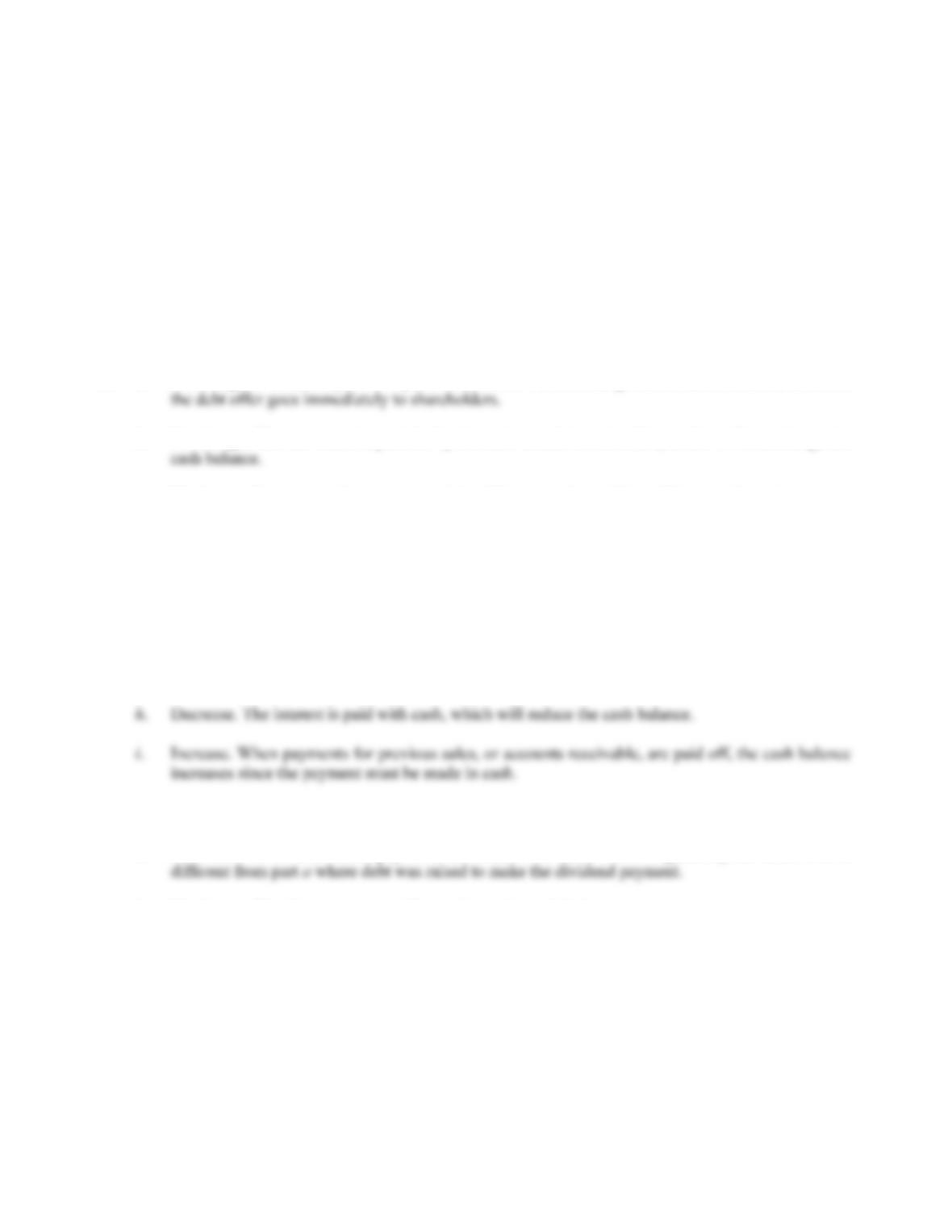B-304 SOLUTIONS
10. BlueSky will need less financing because it is essentially borrowing more from its suppliers. Among
other things, BlueSky will likely need less short-term borrowing from other sources, so it will save on
interest expense.
Solutions to Questions and Problems
NOTE: All end of chapter problems were solved using a spreadsheet. Many problems require multiple steps.
Due to space and readability constraints, when these intermediate steps are included in this solutions
manual, rounding may appear to have occurred. However, the final answer for each problem is found
without rounding during any step in the problem.
Basic
1. a. No change. A dividend paid for by the sale of debt will not change cash since the cash raised from
b. No change. The real estate is paid for by the cash raised from the debt, so this will not change the
c. No change. Inventory and accounts payable will increase, but neither will impact the cash account.
d. Decrease. The short-term bank loan is repaid with cash, which will reduce the cash balance.
e. Decrease. The payment of taxes is a cash transaction.
f. Decrease. The preferred stock will be repurchased with cash.
g. No change. Accounts receivable will increase, but cash will not increase until the sales are paid
off.
j. Decrease. The accounts payable are reduced through cash payments to suppliers.
k. Decrease. Here the dividend payments are made with cash, which is generally the case. This is
l. No change. The short-term note will not change the cash balance.
m. Decrease. The utility bills must be paid in cash.
n. Decrease. A cash payment will reduce cash.
o. Increase. If marketable securities are sold, the company will receive cash from the sale.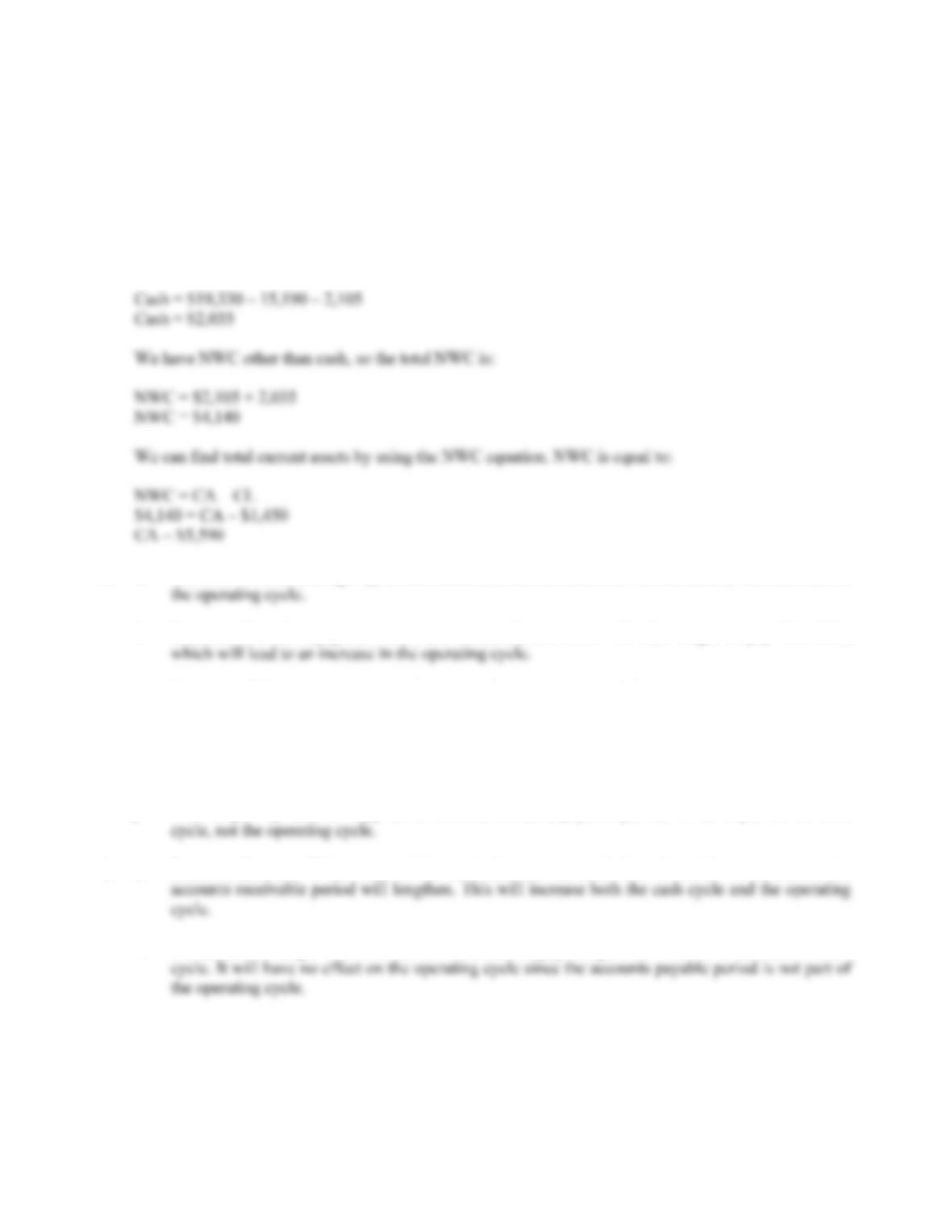CHAPTER 18 B-305
2. The total liabilities and equity of the company are the net book worth, or market value of equity, plus
current liabilities and long-term debt, so:
Total liabilities and equity = \$10,380 + 1,450 + 7,500
Total liabilities and equity = \$19,330
This is also equal to the total assets of the company. Since total assets are the sum of all assets, and cash
is an asset, the cash account must be equal to total assets minus all other assets, so:
3. a. Increase. If receivables go up, the time to collect the receivables would increase, which increases
b. Increase. If credit repayment times are increased, customers will take longer to pay their bills,
c. Decrease. If the inventory turnover increases, the inventory period decreases.
d. No change. The accounts payable period is part of the cash cycle, not the operating cycle.
e. Decrease. If the receivables turnover increases, the receivables period decreases.
f. No change. Payments to suppliers affects the accounts payable period, which is part of the cash
4. a. Increase; Increase. If the terms of the cash discount are made less favorable to customers, the
b. Increase; No change. This will shorten the accounts payable period, which will increase the cash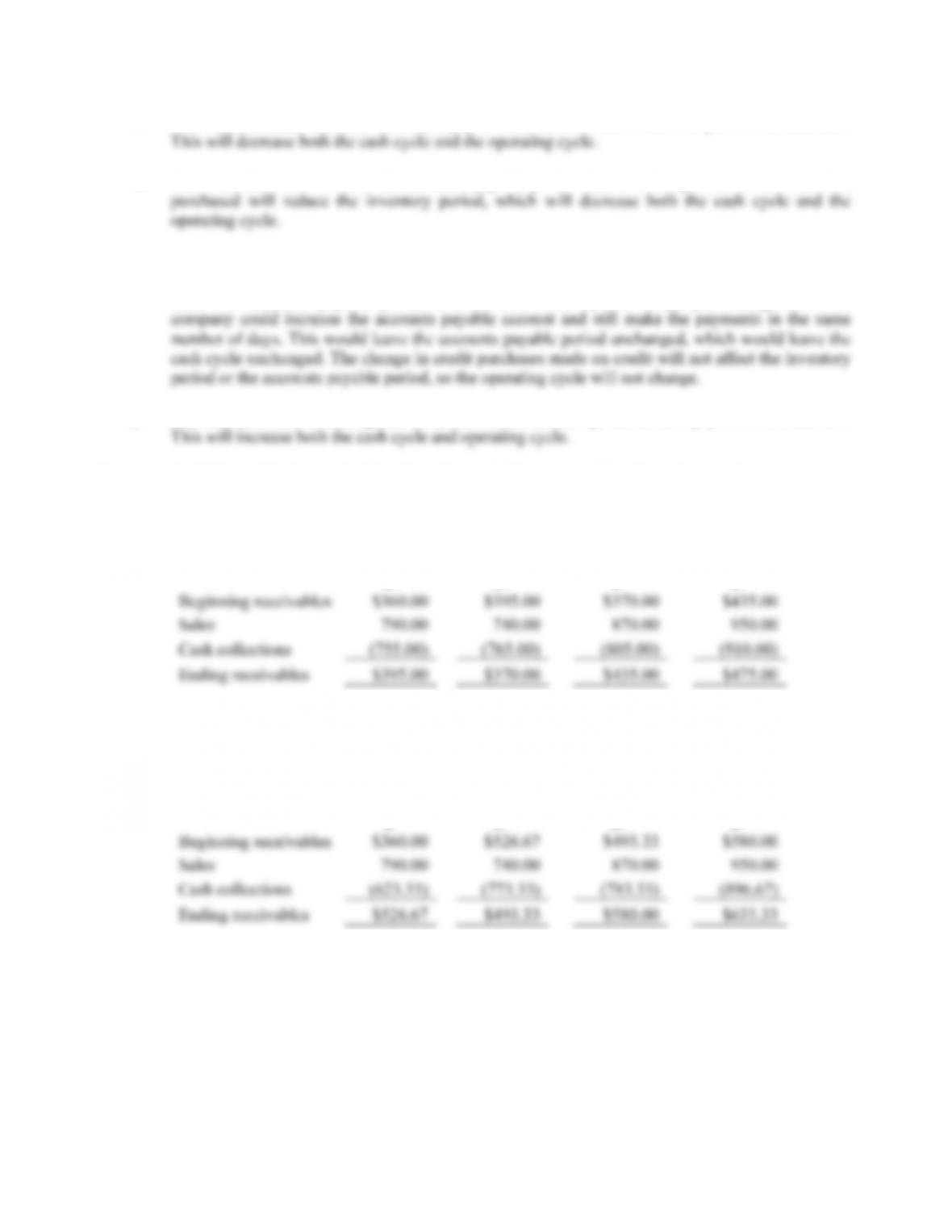B-306 SOLUTIONS
c. Decrease; Decrease. If more customers pay in cash, the accounts receivable period will decrease.
d. Decrease; Decrease. Assume the accounts payable period does not change. Fewer raw materials
e. Decrease; No change. If more raw materials are purchased on credit, the accounts payable period
will tend to increase, which would decrease the cash cycle. We should say that this may not be the
case. The accounts payable period is a decision made by the company’s management. The
f. Increase; Increase. If more goods are produced for inventory, the inventory period will increase.
5. a. A 45-day collection period implies all receivables outstanding from the previous quarter are
collected in the current quarter, and:
(90 – 45)/90 = 1/2 of current sales are collected. So:
Q1 Q2 Q3 Q4
b. A 60-day collection period implies all receivables outstanding from previous quarter are collected
in the current quarter, and:
(90-60)/90 = 1/3 of current sales are collected. So:
Q1 Q2 Q3 Q4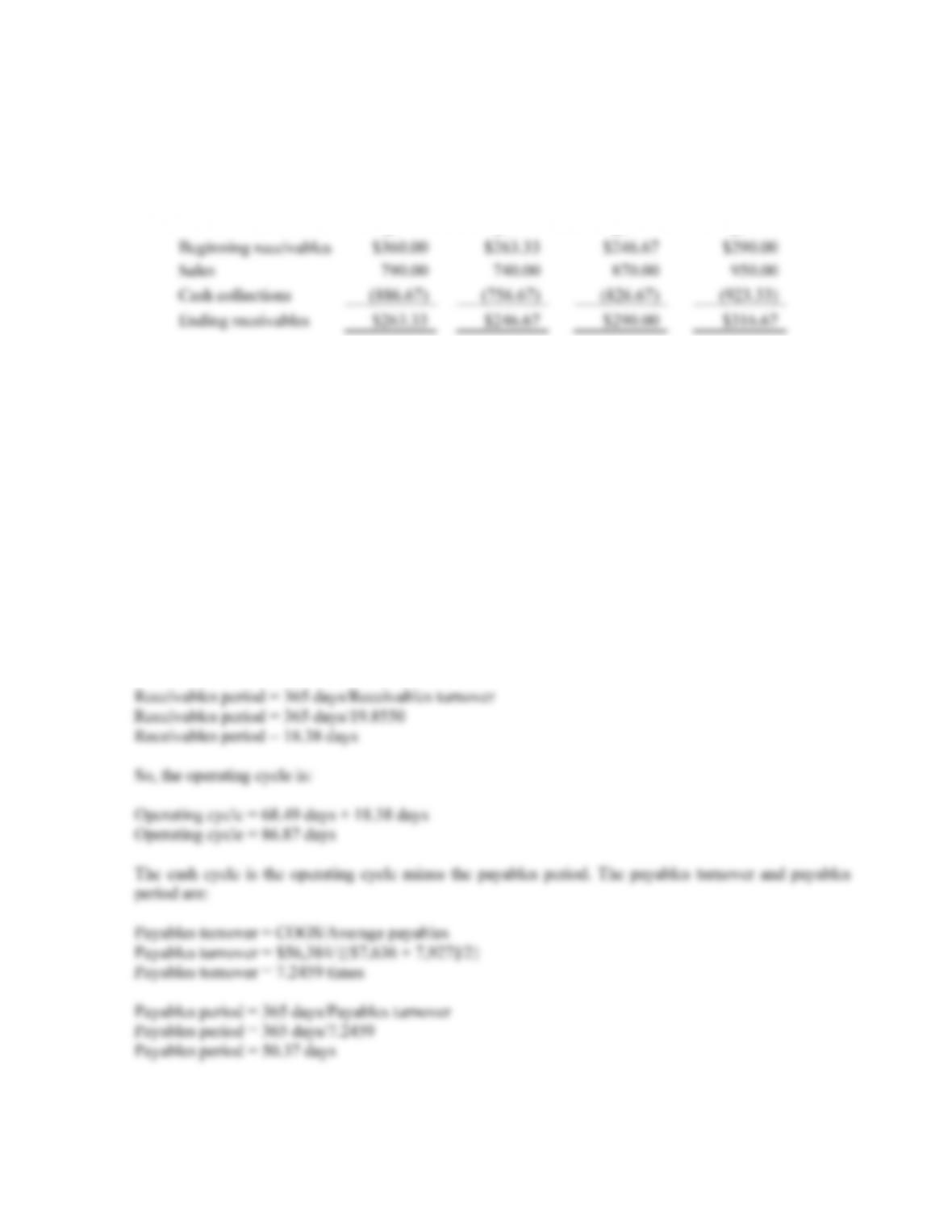CHAPTER 18 B-307
c. A 30-day collection period implies all receivables outstanding from previous quarter are collected
in the current quarter, and:
(90-30)/90 = 2/3 of current sales are collected. So:
Q1 Q2 Q3 Q4
6. The operating cycle is the inventory period plus the receivables period. The inventory turnover and
inventory period are:
Inventory turnover = COGS/Average inventory
Inventory turnover = \$56,384/{[\$9,780 + 11,380]/2}
Inventory turnover = 5.3293 times
Inventory period = 365 days/Inventory turnover
Inventory period = 365 days/5.3293
Inventory period = 68.49 days
And the receivables turnover and receivables period are:
Receivables turnover = Credit sales/Average receivables
Receivables turnover = \$89,804/{[\$4,108 + 4,938]/2}
Receivables turnover = 19.8550 times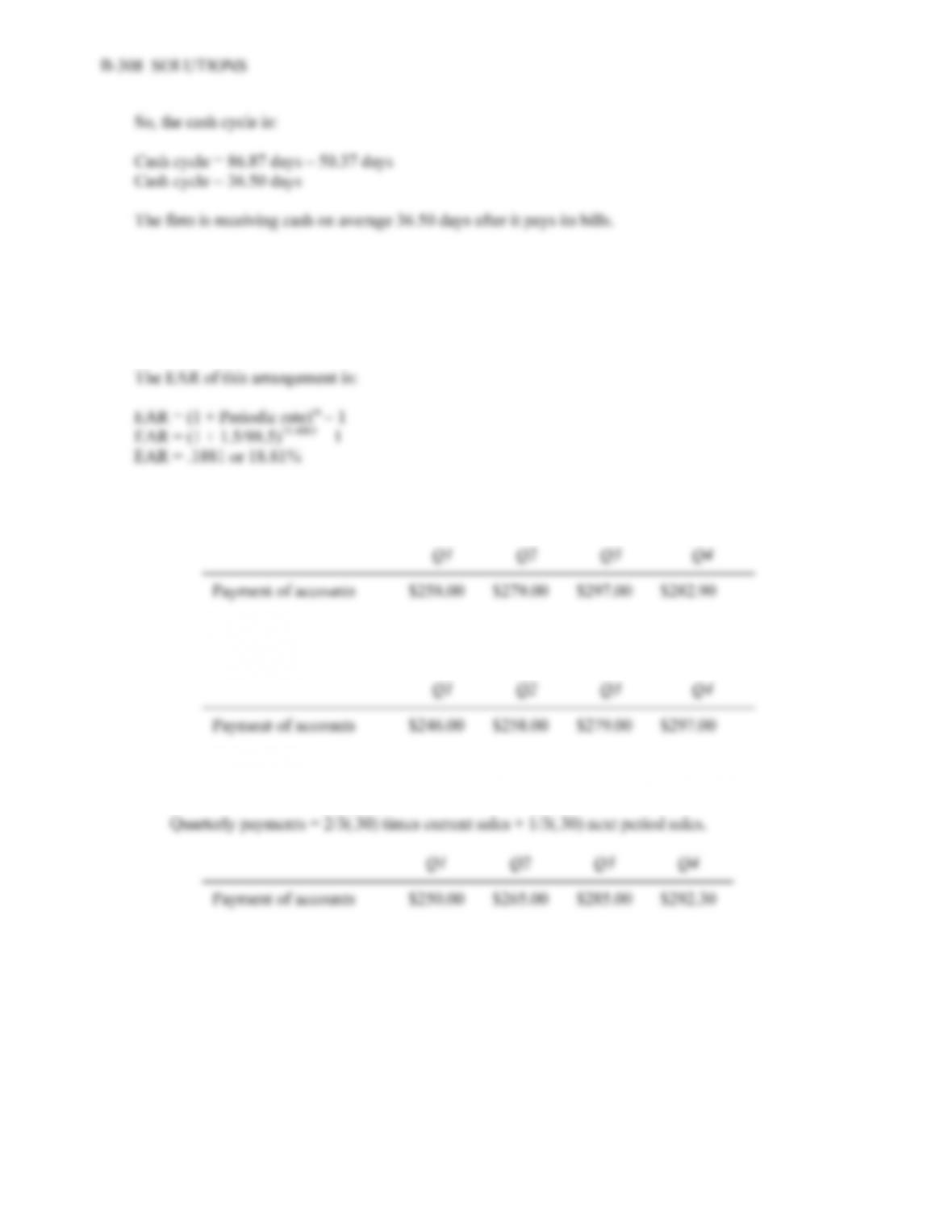7. If we factor immediately, we receive cash on an average of 32 days sooner. The number of periods in a
year are:
Number of periods = 365/32
Number of periods = 11.4063
8. a. The payables period is zero since the company pays immediately. The payment in each period is
30 percent of next period’s sales, so:
b. Since the payables period is 90 days, the payment in each period is 30 percent of the last period’s
sales, so:
c. Since the payables period is 60 days, the payment in each period is 2/3 of last quarter’s orders, plus
1/3 of this quarter’s orders, or: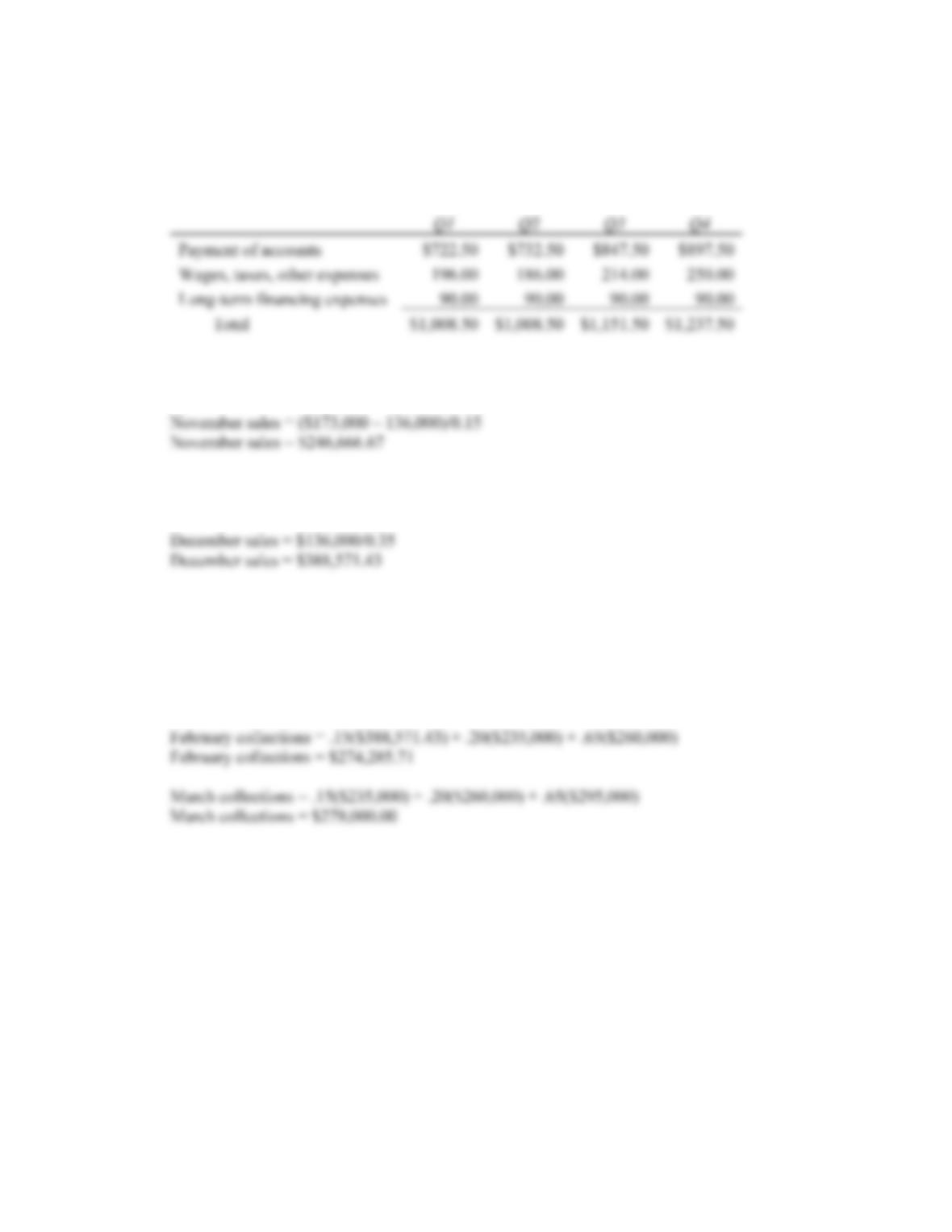CHAPTER 18 B-309
9. Since the payables period is 60 days, the payables in each period will be:
Payables each period = 2/3 of last quarter’s orders + 1/3 of this quarter’s orders
Payables each period = 2/3(.75) times current sales + 1/3(.75) next period sales
10. a. The November sales must have been the total uncollected sales minus the uncollected sales from
December, divided by the collection rate two months after the sale, so:
b. The December sales are the uncollected sales from December divided by the collection rate of the
previous months’ sales, so:
c. The collections each month for this company are:
Collections = .15(Sales from 2 months ago) + .20(Last month’s sales) + .65 (Current sales)
January collections = .15(\$246,666.67) + .20(\$388,571.43) + .65(\$235,000)
January collections = \$267,464.29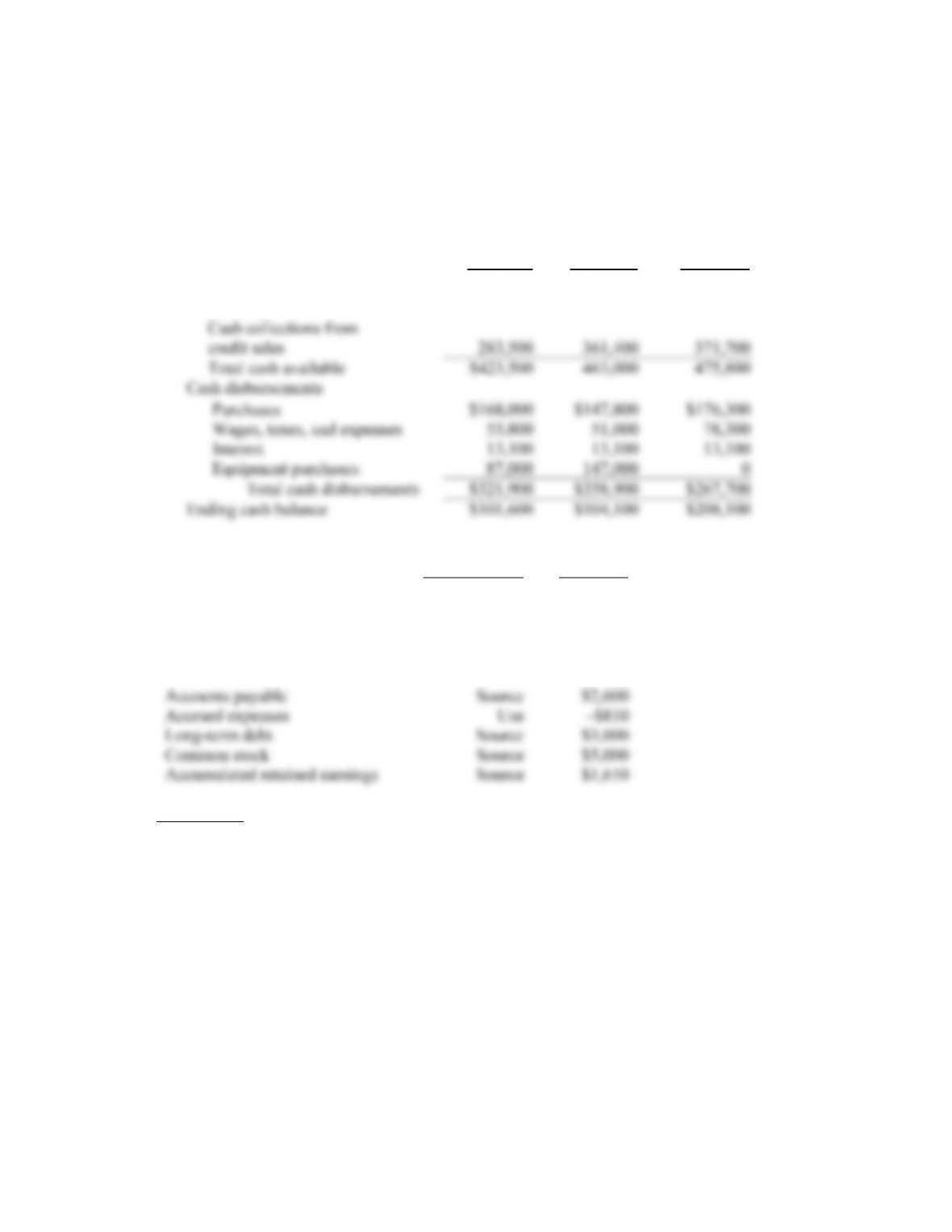B-310 SOLUTIONS
11. The sales collections each month will be:
Sales collections = .35(current month sales) + .60(previous month sales)
Given this collection, the cash budget will be:
April May June
Beginning cash balance \$140,000 \$101,600 \$104,100
Cash receipts
12. Item
Source/Use Amount
Cash Source \$1,100
Accounts receivable Use –\$4,300
Inventories Use –\$3,670
Property, plant, and equipment Use –\$12,720
Intermediate
13. a. If you borrow \$50,000,000 for one month, you will pay interest of:
Interest = \$50,000,000(.0064)
Interest = \$320,000
However, with the compensating balance, you will only get the use of:
Amount received = \$50,000,000 – 50,000,000(.05)